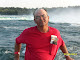# /* // Set the date we're counting down to var countDownDate = new Date("Jan 1, 2019 00:00:00").getTime(); // Update the count down every 1 second var x = setInterval(function() { // Get todays date and time var now = new Date().getTime(); // Find the distance between now and the count down date var distance = countDownDate - now; // Time calculations for days, hours, minutes and seconds var days = Math.floor(distance / (1000 * 60 * 60 * 24)); var hours = Math.floor((distance % (1000 * 60 * 60 * 24)) / (1000 * 60 * 60)); var minutes = Math.floor((distance % (1000 * 60 * 60)) / (1000 * 60)); var seconds = Math.floor((distance % (1000 * 60)) / 1000); // Display the result in the element with id="newyear" document.getElementById("newyear").innerHTML = "There are only " + days + " days until the new year begins!"; //+ hours + "h " + minutes + "m " + seconds + "s "; // If the count down is finished, write some text if (distance < 0) { clearInterval(x); document.getElementById("newyear").innerHTML = "Happy New Year!"; } }, 1000);*/

## Sunday, December 2, 2018

### I think this was our first trip to Utah, probably about 1973, somewhere along I would say Interstate 70, maybe Kansas. The kids on the table are me, my sister Kelli, my nephew Doug, my niece Angie, with my brother Gordon standing by the car